# Expanding Bits in Shrinking Time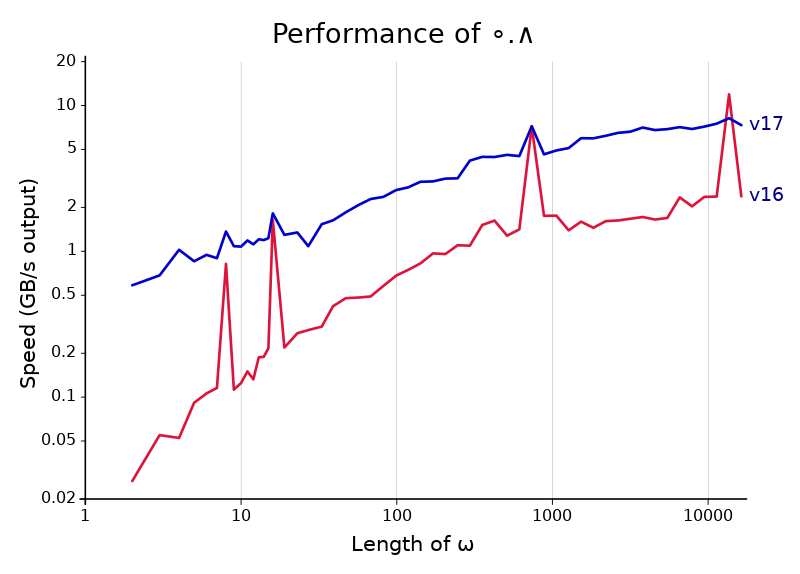The chart above compares the performance of `∘.∧` in Dyalog versions 16.0 and 17.0. The improvement is a factor of 3-4 across the whole range (except at multiple-of-8 lengths, where 16.0 spikes up). That’s not easy to get, especially for a function like `∘.∧` which was already the target of a fair amount of special code. And the improvements are completely general. They apply to any scalar dyadic which has a Boolean result on Booleans, which is all but `+-○`.

How did it happen? The answer, at least for the parts of the graph left of 1024, follows. It takes us through a surprising number of APL primitives, which I think is a great demonstration of the ways APL thinking can lead to faster algorithms even in other languages.

## Binary choices

One of the many performance bumps in Dyalog version 17 is that selecting from an array of two options using a Boolean array is much faster. Dyalog 17 also takes advantage of this speed improvement by using it for a few other functions: scalar dyadics when one argument is a singleton and the other is Boolean, `⍳` (or `∊`) where the right (left) argument is Boolean, and outer products where the left argument is Boolean.

Selection has been sped up for all kinds of indexed array, but the most interesting case is that in which the cells of the indexed array are Boolean, with a cell shape that’s not a multiple of 8 (when working with Boolean algorithms, we tend to call such shapes “odd”). In this case the rows of the result aren’t byte-aligned, so to generate them quickly we will need some Boolean trickery. As it turns out, a lot of Boolean trickery.

The centre of our attention will be the function Replicate (`/`) when the right argument is Boolean and the left is a scalar. That means something like `3/1 0 0 1 0`. Replicate doesn’t seem obviously connected to indexing, but like indexing, it turns each bit of the argument into multiple bits in the result. In fact, this is by far the most important and difficult step in implementing Index, since Index may be phrased straightforwardly in terms of Replicate using exclusive or (`≠`):

``      y[x;]  ←→  (((≢y)/⍪x) ∧⍤1 ≠⌿y) ≠⍤1 ⊣⌿y``

If for some reason that doesn’t look straightforward to you, I’ll explain how it all fits together later. But first, Replicate!

## The trouble with Replicate

Just implementing Replicate on a Boolean array isn’t difficult. Read one bit at a time from the argument, and then write it to the result the required number of times. However, reading and writing one bit at a time is slow. A fast algorithm for Replicate will work an entire machine word at a time (that is, 64 bits on 64-bit systems).

But even fairly well-implemented word-at-a-time strategies will quickly run into another problem: branching. A simple if/then construct in C can be very cheap or very expensive depending on whether the CPU is able to predict in advance which path will be taken. With odd-sized replicates the not-quite-periodic way that the writes interact with byte boundaries will make nearly all useful branching unpredictable. But there are many techniques which can allow the CPU to make choices without having to change the path it takes through a program. Coming up is one rather exotic entry in the field of branchless programming.

## Small expansion factors

When beginning work on fixed-size replicates, I quickly found that, in Dyalog version 16, it was much faster to add a length-1 axis before the first, replicate that, and transpose it to the end than to replicate the last axis directly.

``````      ⎕IO←0 ⋄ x←?1e4⍴2
cmpx '5/⍪x' 'x,,⍨,[0.5]⍨x' '⍉5⌿(1,⍴x)⍴x'
5/⍪x         → 9.5E¯5 |   0% ⎕⎕⎕⎕⎕⎕⎕⎕⎕⎕⎕⎕⎕⎕⎕⎕⎕⎕⎕⎕⎕⎕⎕⎕⎕⎕⎕⎕⎕⎕⎕⎕⎕⎕⎕⎕⎕⎕⎕⎕
x,,⍨,[0.5]⍨x → 3.4E¯5 | -64% ⎕⎕⎕⎕⎕⎕⎕⎕⎕⎕⎕⎕⎕⎕
⍉5⌿(1,⍴x)⍴x  → 1.3E¯5 | -86% ⎕⎕⎕⎕⎕⎕``````

This is largely because I had spent a lot of time improving Transpose with Boolean arguments of various shapes in version 16 (the comparison above looks very different in version 15). Transpose on a short but wide Boolean matrix uses a technique that I call bit interleaving, which would unfortunately require another blog post to explain. To transpose a matrix with r rows, I move across the rows, using some special tricks to insert (r-1) zeroes after each bit in each one, and combine them using bitwise or. To instead replicate a vector by r, I modified this to expand the one argument as though it were going to be combined with other rows, but instead of doing that, I combined it with itself by multiplying by the number whose base-2 representation consists of r ones. (C programmers may notice that multiplying by this number is the same as shifting to the left by r and then subtracting the original value. In fact, using multiplication is faster—x86 variable shifts are slow!)

Bit interleaving is a somewhat complicated algorithm, but it had all been done by the time I started on Replicate, and modifying it didn’t take long.

## Large expansion factors, the obvious way

The bit-interleaving algorithm is very fast for small numbers and gets slower as the expansion factor increases. Above 64 it is no longer usable because it only writes one word at a time, while each bit should expand to more than a word. I chose to stop using it after 32, as it turns out we can get just about the same performance at 33 using a different approach.

The old approach, on the other hand, is only about half as fast at this expansion factor. Version 16’s algorithm reads from the right argument one bit at a time, then writes that bit the appropriate number of times to the result by writing one partial byte and then using memset (which may spill over into the next bit’s area since memset can only write whole bytes; it will be overwritten when we get to the next bit). This works fine for large enough left arguments, and scales up very well: memset is provided by the C standard library and will push bytes to memory as fast as possible. But for small values it is not so good: the call to memset branches a few times based on the unpredictable size of the write, which incurs a steep branch misprediction penalty.

There’s no easy way to get around the penalty for writing some bits at a variable offset. If the expansion factor isn’t a multiple of eight, then the number of bytes each expanded bit touches will always vary by one, and having to check whether to write that byte results in an unpredictable branch. There are ways to avoid a check like this (overwriting is one), but these tend to be fast only on a small range of expansion factors and to be very tricky to write and bug-prone, especially at the boundaries of the result.

## A new idea

So the problem is that writing a weird number of bits at a time is expensive. But it’s possible to get away with only writing one bit for each “memset” we want to perform! The trick is found in a pair of inverse functions well-known for their usefulness in Boolean APL code. These are `{2≠/0,⍵}` and `≠\`, and they are analogous to the pairwise difference `{¯2-/0,⍵}` and progressive sum `+\`. Dyalog 16 has fast code for `≠\` provided by Bob Bernecky, so I knew it was a good building block for other high-performance primitives.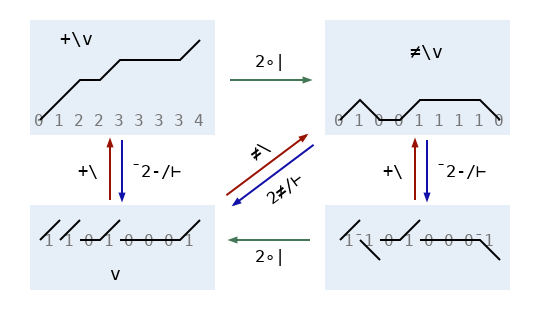The diagram above illustrates the relationships between scans and pairwise differences. In the bitwise world consisting only of even and odd, `≠` is like `+`. But each number is its own negative, so it’s also like `-`. More precisely, since `⍺≠⍵` is the same as `2|⍺+⍵` on Booleans (check for yourself!) and modulus distributes over addition when the final result has a modulus applied to it, `≠\` is equivalent to `2|+\`. Similarly, `{2≠/⍵}` or `{¯2≠/⍵}` is the same as `{2|¯2-/⍵}`. The diagram includes the extra zero in `{2≠/0,⍵}` as part of the vectors in the top half of the diagram, but omits it from the functions used to label arrows to avoid clutter.

Let’s take a look at the pairwise difference after we replicate a vector: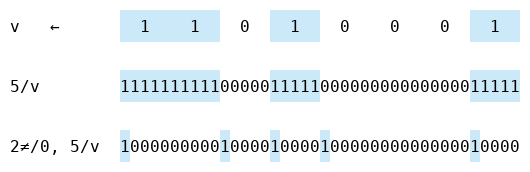It’s very sparse—the only one bits come at indices which are multiples of five. This is because expanding `v` multiplies the indices of its pairwise difference by five:

``````      v←1 1 0 1 0 0 0 1
⍸ 2≠/0, v  ⍝ ⍸⍵  ←→  ⍵/⍳⍴⍵
0 2 3 4 7
⍸ 2≠/0, 5/v
0 10 15 20 35``````

We can recover `5/v` using the inverse `≠\` of pairwise difference. So to expand, we can add four zeros after each bit under the `≠\⍣¯1` function (which is the same as pairwise difference):

``````      ≠\ ,5↑⍪ ≠\⍣¯1⊢ v
1 1 1 1 1 1 1 1 1 1 0 0 0 0 0 1 1 1 1 1 0 0 0 0 0 0 0 0 0 0 0 0 0 0 0 1 1 1 1 1``````

Of course `↑` isn’t particularly fast on these arguments, so we will have to write our own version, which sets the entire result to zero and then writes bits in the appropriate places. Implemented properly, this goes pretty quick, the only substantial costs being a variable shift and one byte-sized write per element on the right. It’s completely branchless. Follow up with the fast `≠\` algorithm and we have a pretty good solution.

This solution performs more writes than it has to, since it writes each difference bit even if it is zero. But trying to get rid of the extra writes is a losing battle. Checking the value before each write takes much longer than just writing it, and even a fancy strategy, like using the count-leading-zeros instruction to quickly skip over all zeros before each one, will be slower for arguments without many zeros in the difference vector. But there is another way to make this algorithm a little faster, coming up after we tie up some loose ends…

## Back to Index, and more xor

What about my unexplained formula for indexing? The expression

``      y[x;]  ←→  (((≢y)/⍪x) ∧⍤1 ≠⌿y) ≠⍤1 ⊣⌿y``

also relies on insight about `≠`, but in a completely different context. Let’s simplify it a bit to see what’s going on. Suppose we have two Boolean arrays `b` and `c` of the same shape, and we want to use the bit `a` to select one of them: we want an expression that returns `b` if `a=0` and `c` if `a=1`. For real numbers, we might use a linear combination:

``      b + a×(c-b)``

If `a=0`, then the `c-b` term is removed and we get `b`. If `a=1`, then it stays and we have `(b+(c-b)) = c`.
This formula is also valid for Boolean arrays, of course, but introducing an integer `c-b` would be a poor choice from a performance standpoint. Instead, we can use the insight that `≠` performs the function of both `+` and `-` in the bitwise world, and transliterate our formula into

``      b ≠ a∧(c≠b)``

Again, if `a=0`, then the `c≠b` is zeroed out, and we end up with `b≠0`, which is the same as `b`. If `a=1`, then it stays, leaving `(b≠(c≠b))`, which gives `c`.

If `y` is a shape `2 m` array `y←b,[¯0.5]c`, then we have `b ≡ ⊣⌿y` and `(c≠b) ≡ ≠⌿y`. So `b ≠ a∧(c≠b)` is, rearranging some, the same as `(a ∧ ≠⌿y) ≠ ⊣⌿y`. But there is an implicit scalar extension in the `∧` function; we can make this explicit by writing `((m/a) ∧ ≠⌿y) ≠ ⊣⌿y`. Now it’s clear that to select using each bit of a vector `x`, we need to turn each bit into a row with length `m`, and then apply the `∧` and `≠` functions on rows, giving the formula I showed earlier.

How about Outer Product? That one’s easier, with no Boolean magic required. For vector arguments, `⍺ ∘.f ⍵` is identical to `((≢⍵)/⍪⍺) f ((≢⍺),≢⍵)⍴⍵`, and the same computation works for general arrays if we use the ravel length `×/∘⍴` instead of the count `≢`. When the right argument has more than around 1024 bits, replicate becomes slower than the old way of just pairing each bit of the left argument with the whole of the right, so we use that method instead.

## Speeding up ≠\

We left off with an expansion algorithm whose cost is dominated by the time to compute `≠\` on a vector. That computation is based on a fast method for `≠\` on a single machine word. It moves along the argument vector (which is the same as the result vector in this case) one word at a time, keeping track of the most recent bit of the result. Then each word of the result is obtained from the fast xor-scan of that word in the argument, xor-ed with the carried bit. For arbitrary input vectors, this is the best algorithm. But the word-length `≠\` computation is slowing us down, and it turns out we can do a lot better.

Writing a single bit has the same cost as writing an entire aligned machine word. So writing one bit at a time, while much better than writing a variable number of bits with arbitrary alignment, represents some wasted effort. Can we make the impending `≠\` computation easier by using full-word writes?

A definite “yes” for that question. In fact, we can eliminate all of the intra-word computation. Consider the effect of a bit that we write on the final result after applying `≠\`. That bit will be spread out over all of the subsequent bits, flipping each one. In order to produce this effect on a single word (leaving the task of flipping subsequent words for later), we can thus xor an aligned word with a word which is zero before that bit and one at that bit and later. We can get this word just by shifting the all-ones word right by an appropriate amount. And then we can omit the expensive word-size `≠\` computation entirely, so that to perform the final pass we simply repeatedly xor the next word with the current one’s last bit. This greatly reduces the cost of the final pass at very little expense to the pass before it (xor-ing rather than setting a word requires reading that word’s value first, so it’s not quite free).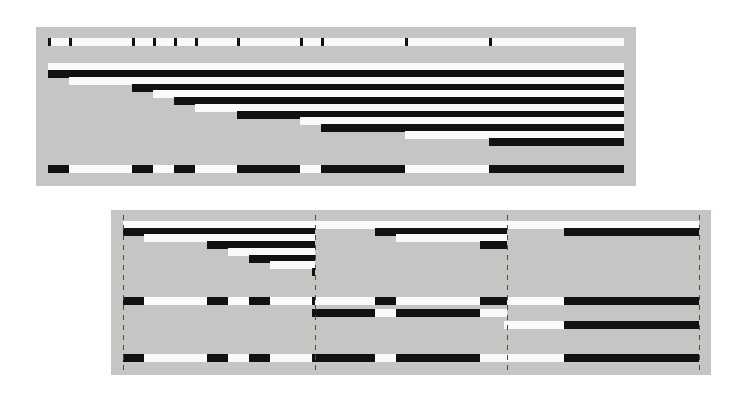To summarize, there are three passes:

• Set all bits of the result to zero.
• For each bit in the right argument, xor the appropriate word in the result with a word which is all ones after the place where that bit starts in the result.
• Iterate across words of the result, xor-ing each with the last bit of the previous word (after finishing computation on that word).

For expansion factors between 32 and 256, this is the fastest method I know of. Below 32, interleaving is faster, and above 256 simply using memset will do the trick.

The xor-based algorithm also works for Replicate or Expand when the left argument is a vector, and in Dyalog 17 it is used for both of these whenever the average expansion factor, which is equal to the ratio of result length to argument length, is 256 or less.

## The end result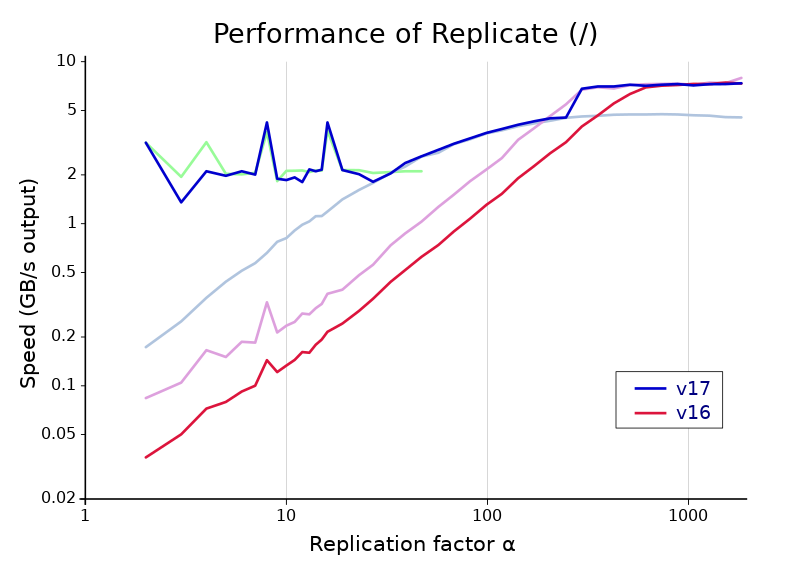The graph above shows the gains in scalar-Boolean replicate between versions 16.0 and 17.0. Note the huge scale of the vertical axis—replicate is just short of a hundred times faster at the far left! Version 17’s Replicate is split into three parts, with the performance of each individual algorithm shown under the overall result. From left to right, these are the algorithm with bit interleaving, the xor-based algorithm described in the last section, and an algorithm with memset. The last of these is the same basic strategy used by version 16, but there are some improvements in version 17 that make it about 45% faster for short arguments.

The seams between these algorithms are not quite perfect on my machine, but they are not off by enough to damage performance significantly. The cutoffs favor the middle, xor-based algorithm a little. I think this is appropriate because that algorithm is likely to have consistent performance even on older machines and different architectures. In contrast, bit interleaving uses the BMI2 instruction set (available on x86 processors since 2013) and is slower if it is not present, and memset may perform differently with other implementations of the standard library or depending on available vector instruction sets. Our xor-based algorithm uses only very widely available instructions, and one of the costs—the variable shift used on the all-ones word—is actually much faster on other architectures because of a design flaw in x86. An excellent addition to Dyalog APL!